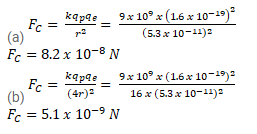# The average separation between the proton and the electronQuestion:

The average separation between the proton and the electron in a hydrogen atom in ground state is $5.3 \times 10^{-11} \mathrm{~m}$.

(a) Calculate the Coulomb force between them at this separation

(b) When the atom goes into its first excited state the average separation between the proton and the electron increases to four times its value in the ground state. What is the Coulomb force in this state?

Solution: